# Selina Solutions Concise Mathematics Class 6 Chapter 12: Proportion Exercise 12(C)

Selina Solutions Concise Mathematics Class 6 Chapter 12 Proportion Exercise 12(C), help students with clear answers about the concepts covered here, according to the latest syllabus. To determine that the given numbers are in proportion, is the main topic discussed under this exercise. Students who aspire to score well in the annual examination, are suggested to practice Selina Solutions thoroughly on a daily basis. In order to build a stronghold on the concepts, Selina Solutions Concise Mathematics Class 6 Chapter 12 Proportion Exercise 12(C) PDF, can be downloaded from the links, which are available below

## Selina Solutions Concise Mathematics Class 6 Chapter 12: Proportion Exercise 12(C) Download PDF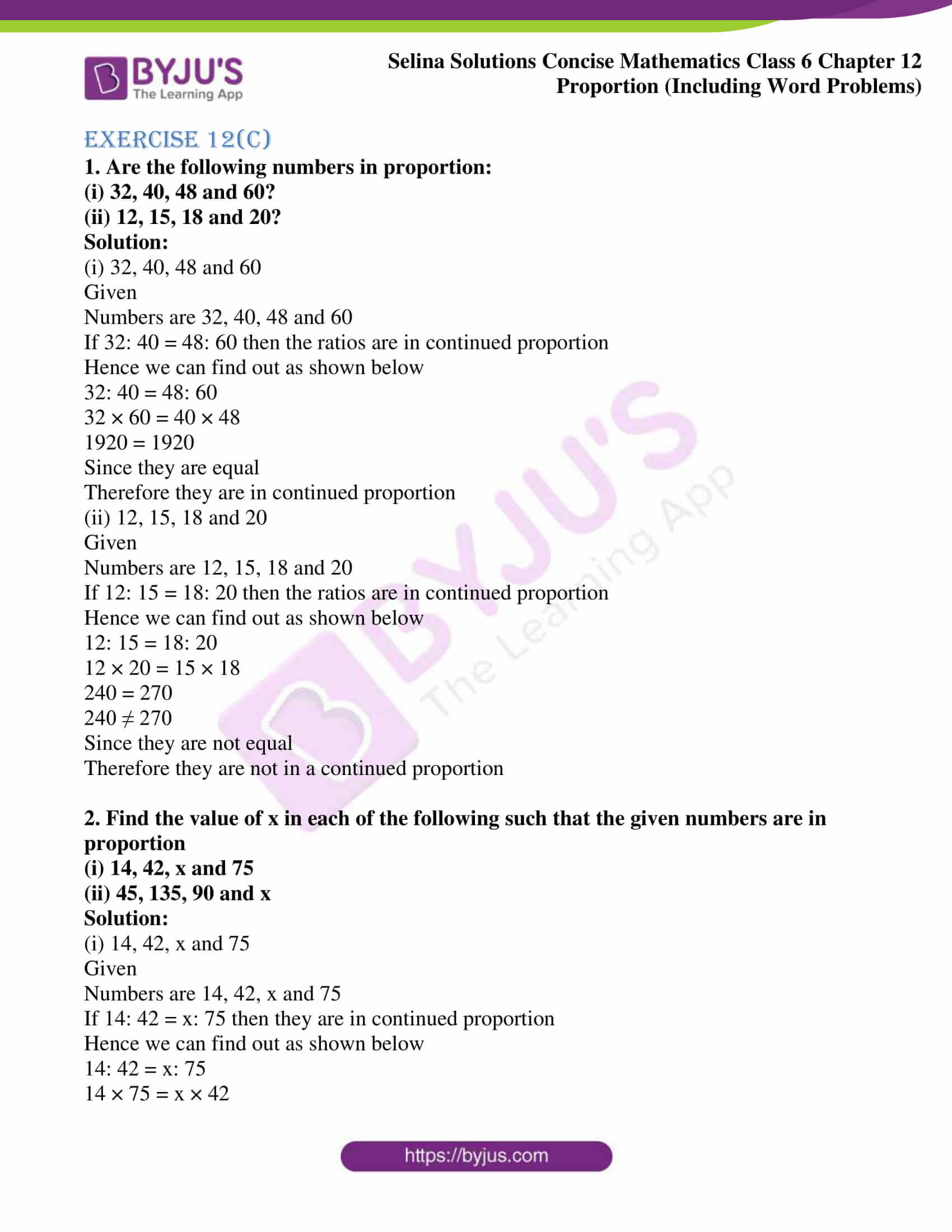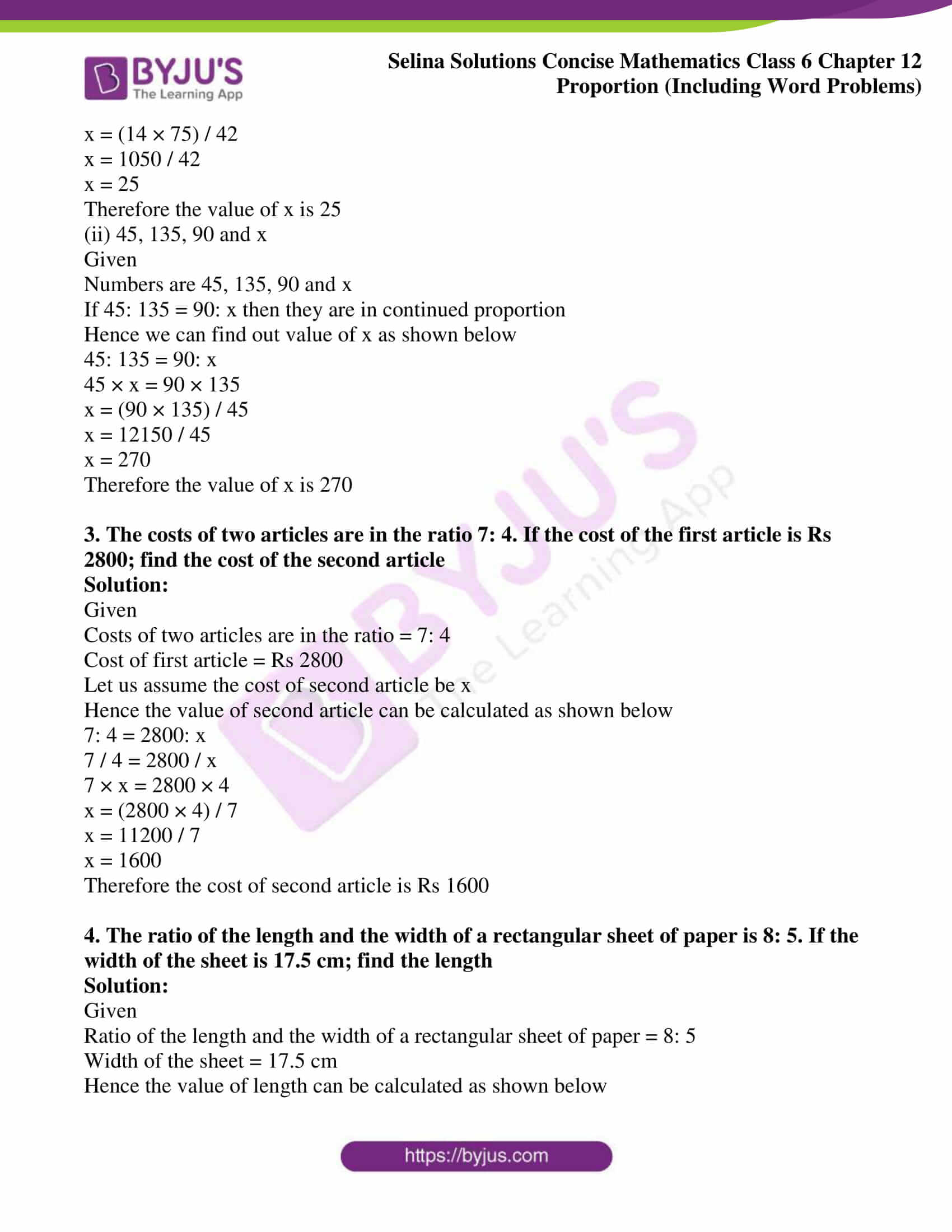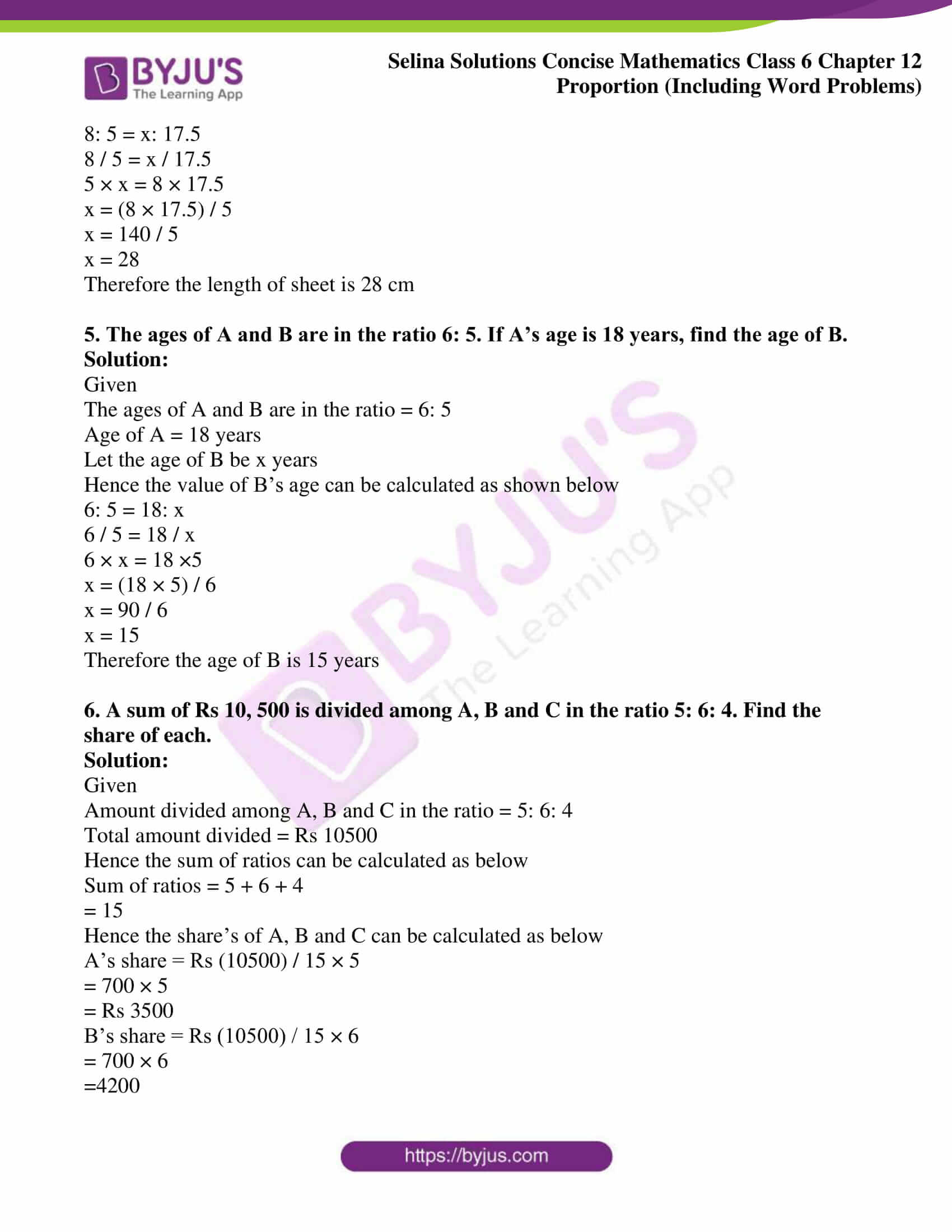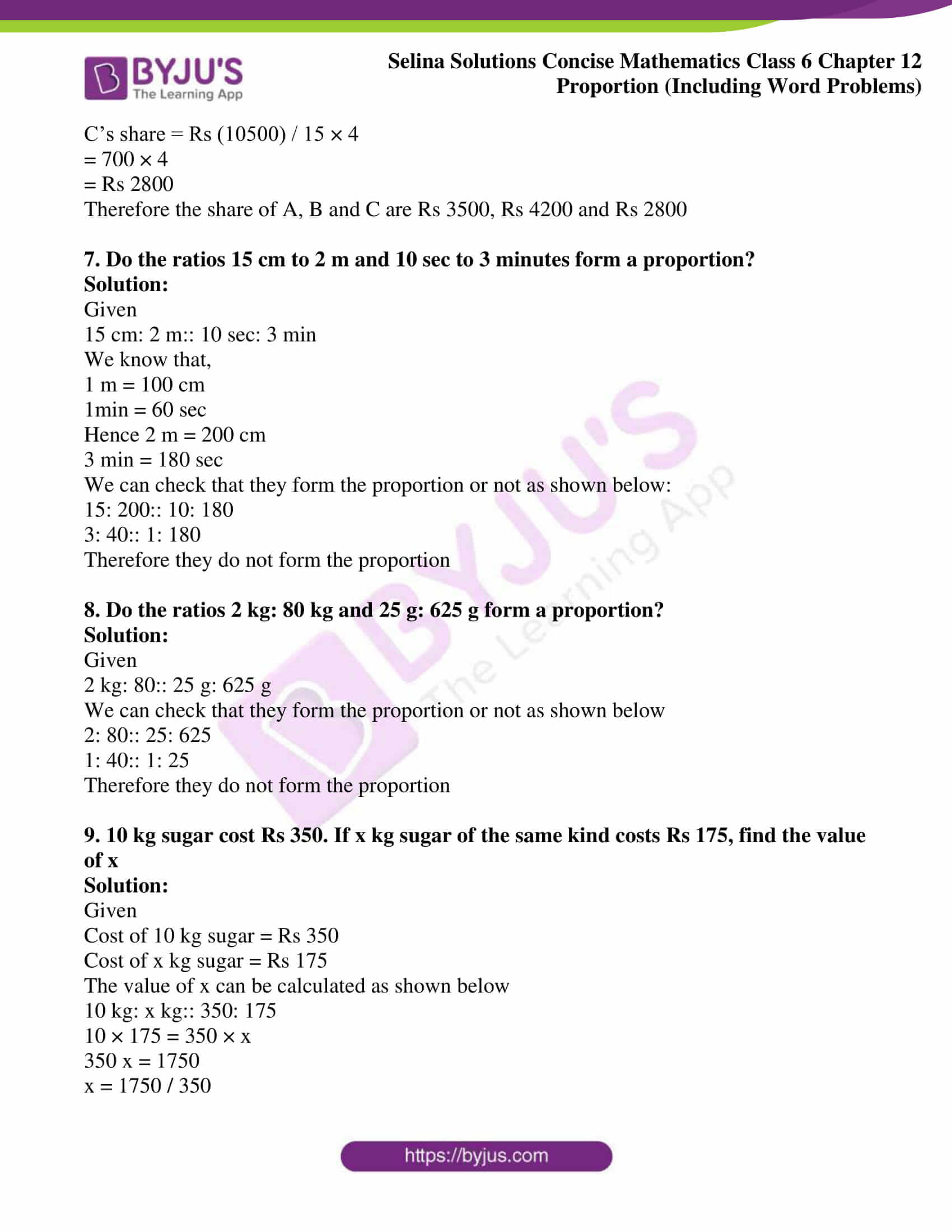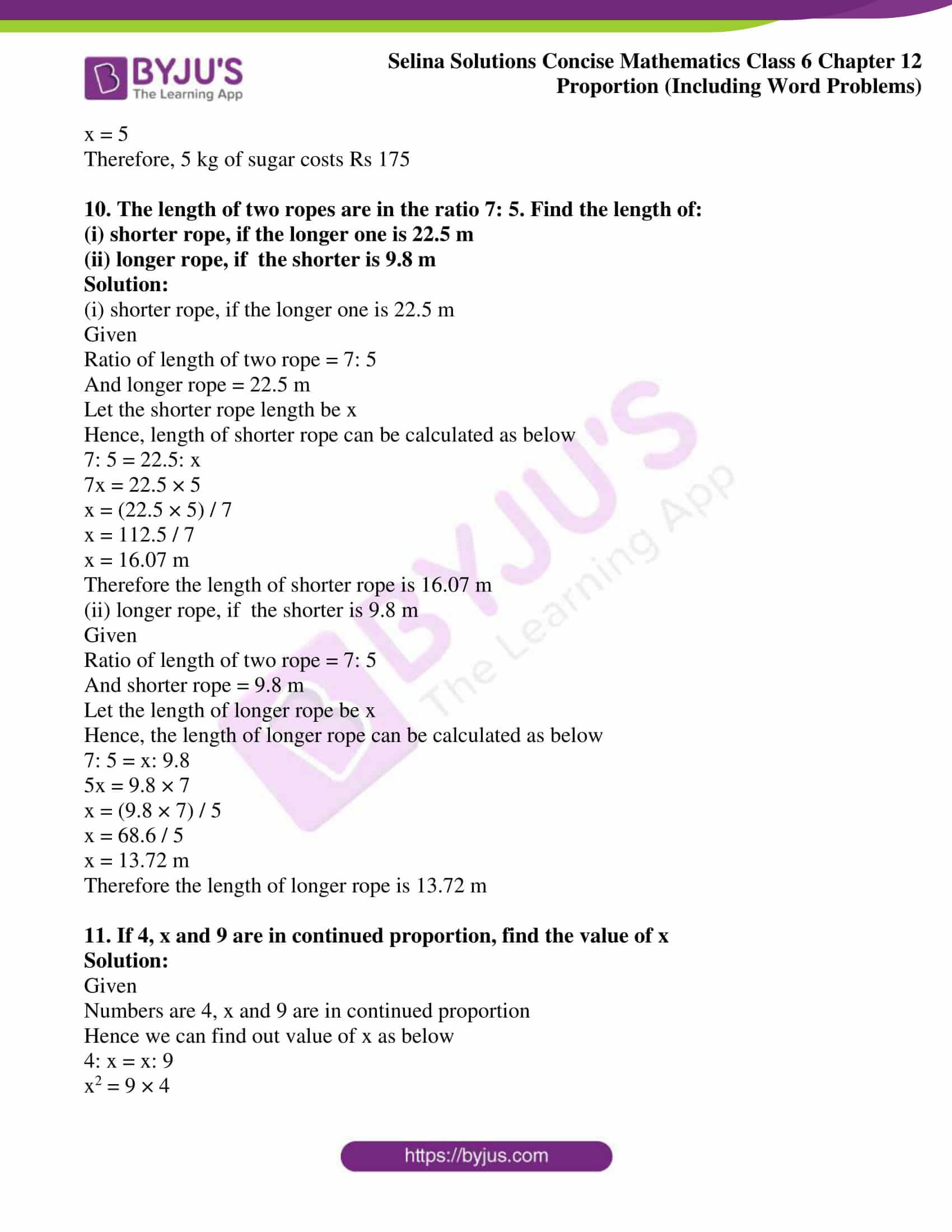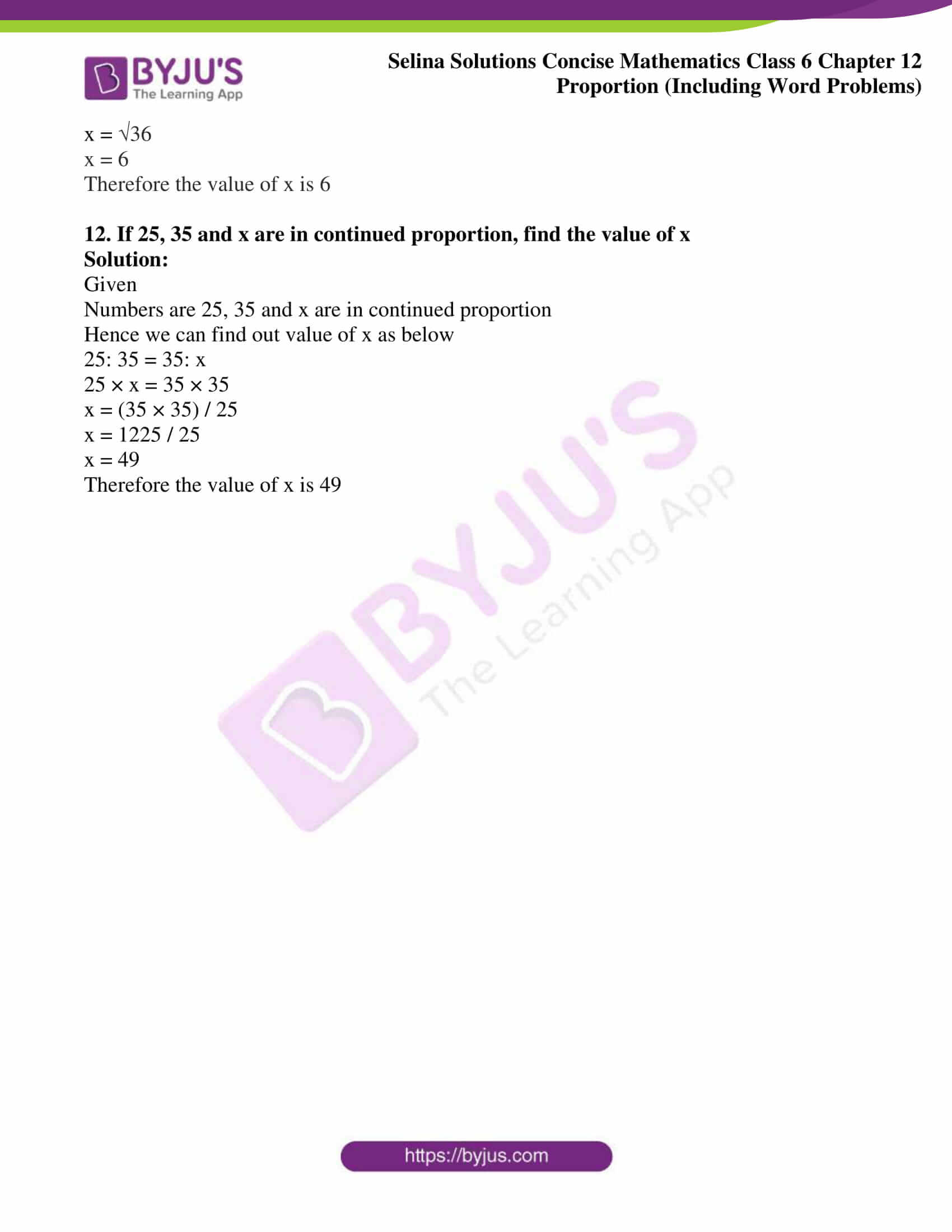### Access other exercises of Selina Solutions Concise Mathematics Class 6 Chapter 12: Proportion

Exercise 12(A) Solutions

Exercise 12(B) Solutions

### Access Selina Solutions Concise Mathematics Class 6 Chapter 12: Proportion Exercise 12(C)

Exercise 12(C)

1. Are the following numbers in proportion:

(i) 32, 40, 48 and 60?

(ii) 12, 15, 18 and 20?

Solution:

(i) 32, 40, 48 and 60

Given

Numbers are 32, 40, 48 and 60

If 32: 40 = 48: 60 then the ratios are in continued proportion

Hence we can find out as shown below

32: 40 = 48: 60

32 × 60 = 40 × 48

1920 = 1920

Since they are equal

Therefore they are in continued proportion

(ii) 12, 15, 18 and 20

Given

Numbers are 12, 15, 18 and 20

If 12: 15 = 18: 20 then the ratios are in continued proportion

Hence we can find out as shown below

12: 15 = 18: 20

12 × 20 = 15 × 18

240 = 270

240 ≠ 270

Since they are not equal

Therefore they are not in a continued proportion

2. Find the value of x in each of the following such that the given numbers are in proportion

(i) 14, 42, x and 75

(ii) 45, 135, 90 and x

Solution:

(i) 14, 42, x and 75

Given

Numbers are 14, 42, x and 75

If 14: 42 = x: 75 then they are in continued proportion

Hence we can find out as shown below

14: 42 = x: 75

14 × 75 = x × 42

x = (14 × 75) / 42

x = 1050 / 42

x = 25

Therefore the value of x is 25

(ii) 45, 135, 90 and x

Given

Numbers are 45, 135, 90 and x

If 45: 135 = 90: x then they are in continued proportion

Hence we can find out value of x as shown below

45: 135 = 90: x

45 × x = 90 × 135

x = (90 × 135) / 45

x = 12150 / 45

x = 270

Therefore the value of x is 270

3. The costs of two articles are in the ratio 7: 4. If the cost of the first article is Rs 2800; find the cost of the second article

Solution:

Given

Costs of two articles are in the ratio = 7: 4

Cost of first article = Rs 2800

Let us assume the cost of second article be x

Hence the value of second article can be calculated as shown below

7: 4 = 2800: x

7 / 4 = 2800 / x

7 × x = 2800 × 4

x = (2800 × 4) / 7

x = 11200 / 7

x = 1600

Therefore the cost of second article is Rs 1600

4. The ratio of the length and the width of a rectangular sheet of paper is 8: 5. If the width of the sheet is 17.5 cm; find the length

Solution:

Given

Ratio of the length and the width of a rectangular sheet of paper = 8: 5

Width of the sheet = 17.5 cm

Hence the value of length can be calculated as shown below

8: 5 = x: 17.5

8 / 5 = x / 17.5

5 × x = 8 × 17.5

x = (8 × 17.5) / 5

x = 140 / 5

x = 28

Therefore the length of sheet is 28 cm

5. The ages of A and B are in the ratio 6: 5. If A’s age is 18 years, find the age of B.

Solution:

Given

The ages of A and B are in the ratio = 6: 5

Age of A = 18 years

Let the age of B be x years

Hence the value of B’s age can be calculated as shown below

6: 5 = 18: x

6 / 5 = 18 / x

6 × x = 18 ×5

x = (18 × 5) / 6

x = 90 / 6

x = 15

Therefore the age of B is 15 years

6. A sum of Rs 10, 500 is divided among A, B and C in the ratio 5: 6: 4. Find the share of each.

Solution:

Given

Amount divided among A, B and C in the ratio = 5: 6: 4

Total amount divided = Rs 10500

Hence the sum of ratios can be calculated as below

Sum of ratios = 5 + 6 + 4

= 15

Hence the share’s of A, B and C can be calculated as below

A’s share = Rs (10500) / 15 × 5

= 700 × 5

= Rs 3500

B’s share = Rs (10500) / 15 × 6

= 700 × 6

=4200

C’s share = Rs (10500) / 15 × 4

= 700 × 4

= Rs 2800

Therefore the share of A, B and C are Rs 3500, Rs 4200 and Rs 2800

7. Do the ratios 15 cm to 2 m and 10 sec to 3 minutes form a proportion?

Solution:

Given

15 cm: 2 m:: 10 sec: 3 min

We know that,

1 m = 100 cm

1min = 60 sec

Hence 2 m = 200 cm

3 min = 180 sec

We can check that they form the proportion or not as shown below:

15: 200:: 10: 180

3: 40:: 1: 180

Therefore they do not form the proportion

8. Do the ratios 2 kg: 80 kg and 25 g: 625 g form a proportion?

Solution:

Given

2 kg: 80:: 25 g: 625 g

We can check that they form the proportion or not as shown below

2: 80:: 25: 625

1: 40:: 1: 25

Therefore they do not form the proportion

9. 10 kg sugar cost Rs 350. If x kg sugar of the same kind costs Rs 175, find the value of x

Solution:

Given

Cost of 10 kg sugar = Rs 350

Cost of x kg sugar = Rs 175

The value of x can be calculated as shown below

10 kg: x kg:: 350: 175

10 × 175 = 350 × x

350 x = 1750

x = 1750 / 350

x = 5

Therefore, 5 kg of sugar costs Rs 175

10. The length of two ropes are in the ratio 7: 5. Find the length of:

(i) shorter rope, if the longer one is 22.5 m

(ii) longer rope, if the shorter is 9.8 m

Solution:

(i) shorter rope, if the longer one is 22.5 m

Given

Ratio of length of two rope = 7: 5

And longer rope = 22.5 m

Let the shorter rope length be x

Hence, length of shorter rope can be calculated as below

7: 5 = 22.5: x

7x = 22.5 × 5

x = (22.5 × 5) / 7

x = 112.5 / 7

x = 16.07 m

Therefore the length of shorter rope is 16.07 m

(ii) longer rope, if the shorter is 9.8 m

Given

Ratio of length of two rope = 7: 5

And shorter rope = 9.8 m

Let the length of longer rope be x

Hence, the length of longer rope can be calculated as below

7: 5 = x: 9.8

5x = 9.8 × 7

x = (9.8 × 7) / 5

x = 68.6 / 5

x = 13.72 m

Therefore the length of longer rope is 13.72 m

11. If 4, x and 9 are in continued proportion, find the value of x

Solution:

Given

Numbers are 4, x and 9 are in continued proportion

Hence we can find out value of x as below

4: x = x: 9

x2 = 9 × 4

x = 36

x = 6

Therefore the value of x is 6

12. If 25, 35 and x are in continued proportion, find the value of x

Solution:

Given

Numbers are 25, 35 and x are in continued proportion

Hence we can find out value of x as below

25: 35 = 35: x

25 × x = 35 × 35

x = (35 × 35) / 25

x = 1225 / 25

x = 49

Therefore the value of x is 49